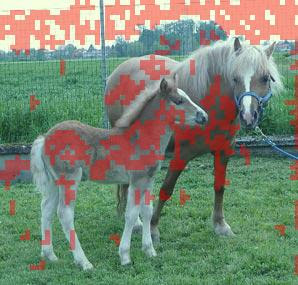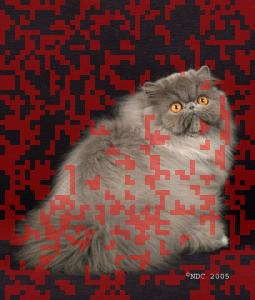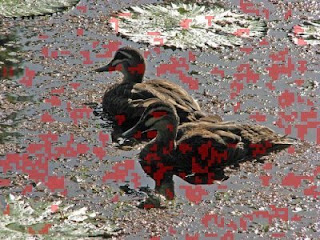## Sunday, September 14, 2008

### Detecting similar entropy zones in image

As I thought about information entropy one idea came to me - to write application which looks similar entropy zones in image. So after some time, I came with this algorithm (pseudo code):

1. Split image into 5x5 pixel image blocks
2. Calculate information entropy of these blocks (actually sum of entropy in 3 color channels)
3. Find similar entropy blocks.
4. [...] filter out small groups of blocks (seems like noise, huh ? ). Blah, blah...
5. Picture these similar entropy blocks on top of original image as red color layer.

Now real part (as you know already) - Python code which does the job [you need PIL module to run this]:

`from PIL import Imagefrom math import *def entropysum(pixels): """ Calculating information entropy for image region and returning entropy sum for all 3 color channels """ cr = [r for (r,g,b) in pixels] cg = [g for (r,g,b) in pixels] cb = [b for (r,g,b) in pixels]  er = 0.0 eg = 0.0 eb = 0.0  for r in set(cr):  p = float(cr.count(r))/len(cr)  if p > 0.0: er += -p * log(p,2) for g in set(cg):  p = float(cg.count(g))/len(cg)  if p > 0.0: eg += -p * log(p,2) for b in set(cb):  p = float(cb.count(b))/len(cb)  if p > 0.0: eb += -p * log(p,2) return er + eg + ebdef decompose(image, block_len): """ Decomposing given image into some number of smaller images of size block_len*block_len """ parts = [] w, h = image.size  for x in range(0, w, block_len):  for y in range(0, h, block_len):   locim = image.crop((x,y,x+block_len,y+block_len))   acc = entropysum(list(locim.getdata()))   parts.append((acc,x,y,locim))  parts.sort()  return partsdef similarparts(imagparts): """ Detecting similar image blocks by comparing entropy of given images. Two images considered being equal if entropy difference is not big. """ dupl = []  for i in range(len(imagparts)-1):  acc1, x1, y1, im1 = imagparts[i]  acc2, x2, y2, im2 = imagparts[i+1]    if acc1 == acc2 == 0:   gain = 0.0  else:   gain = 100.0 * (1.0 - acc1 / acc2)  if 0.01 < gain < 0.1 :   if imagparts[i] not in dupl:    dupl.append(imagparts[i])   if imagparts[i+1] not in dupl:    dupl.append(imagparts[i+1]) return dupldef clusterparts(parts): """ Grouping nearest images into groups. This is done, because we need to filter out very small groups. We want to know only big differences. """  filtparts = [] clust = {} belongs = {} w,h = parts.size  # assign all parts to clusters for i in range(len(parts)):  acc, x, y, im = parts[i]  sides = []  sides.append(str(x)+str(y)+str(x+w)+str(y))  sides.append(str(x+w)+str(y)+str(x+w)+str(y+h))  sides.append(str(x)+str(y+h)+str(x+w)+str(y+h))  sides.append(str(x)+str(y)+str(x)+str(y+h))    # detect side already in cluster  fc = None  for s in sides:   if belongs.has_key(s):    fc = belongs[s]    break    # if this is new cluster  if fc == None:   fc = len(clust) + 1   clust[fc] = 1  else:   clust[fc] += 1    # set cluster for rectangle sides  for s in sides:   if not belongs.has_key(s):    belongs[s] = fc # filter out small clusters for i in range(len(parts)):  acc, x, y, im = parts[i]  side = str(x)+str(y)+str(x+w)+str(y)  cl = belongs[side]  if clust[cl] > 2:   filtparts.append(parts[i])  return filtpartsdef marksimilar(image, dparts): """ Mark found similar image blocks on original image, by applying red layer on similar parts of image. """ if dparts:  colormask = Image.new('RGB', dparts.size,(255,0,0))  for (acc,x,y,im) in dparts:   im = Image.blend(im, colormask, 0.4)   image.paste(im,(x,y))  return imageif __name__ == '__main__': im = Image.open("1.jpg") ls = decompose(im, 5) dparts = similarparts(ls) cparts = clusterparts(dparts) im = marksimilar(im, cparts) im.show()`

So these are the results after running this script on several images:Conclusion

So this algorithm is an interesting tool for exploration of information entropy in image. Maybe in some cases it could be a tool for analyzing very similar texture zones. BTW information entropy may be used for hashing image. Hashing image is useful, because it lets us to search similar images in database (for example) by its hash.

Have fun !